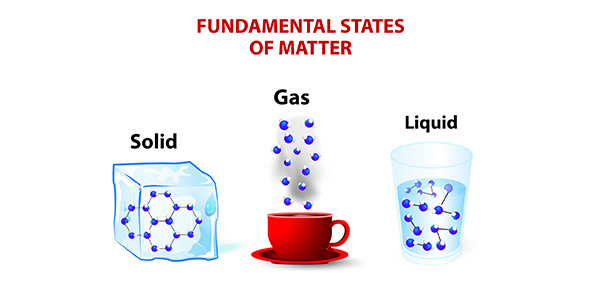# 69a Measuring Matter

10 Questions | Total Attempts: 34SettingsRelated Topics
• 1.
All matter has _____?
• A.

Objects

• B.

Mass

• C.

Shape

• 2.
The mass of an object is _____?
• A.

The amount of matter it has.

• B.

The type of matter it has.

• C.

How much space it takes up.

• 3.
Volume is _____?
• A.

How much matter an object has

• B.

How much space an object takes up

• C.

How much mass an object has

• 4.
The volume of a box is _____?
• A.

Its length plus its width plus its heigh

• B.

Its length times its width times its height

• C.

Its length times its width plus its height

• 5.
The volume of a liquid in a beaker is the number _____.
• A.

At the top of the beaker

• B.

At the bottom of the beaker

• C.

On the beaker next to the top surface of the liquid

• 6.
The volume of a solid placed in a beaker of liquid is the _____.
• A.

Volume of the liquid and solid minus the volume of the liquid

• B.

Volume of the liquid and solid plus the volume of the liquid

• C.

Volume of the liquid multiplied by the volume of the liquid

• 7.
Mass is measured in _____.
• 8.
The _____ will tell you how much space the box takes up.
• 9.
The volume of a liquid can be calculated in milliliters or _____.
• 10.
_____ is a measure of size, or how much space an object takes up.